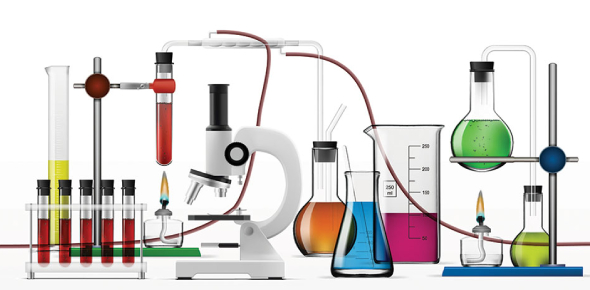# Physical Chemistry Trivia Quiz!

14 Questions | Total Attempts: 33Settings• 1.
The process in which substance gains electrons is called
• A.

Reduction

• B.

Sublimation

• C.

Hydrogenation

• D.

Oxidation

• 2.
An electrolytic cell uses electrical energy to drive
• A.

Biochemical reaction

• B.

None

• C.

Chemical reaction

• D.

No reaction

• 3.
Loss of hydrogen atoms by an element is called
• A.

Reduction

• B.

Oxidation

• C.

Hydrogenation

• D.

Sublimation

• 4.
The elements whose oxidation number is increased are:
• A.

Reduced

• B.

Hydrogenated

• C.

None

• D.

Oxidized

• 5.
An electrochemical cell is also called
• A.

Battery cell

• B.

Galvanic cell

• C.

Cell

• D.

Chargeable cell

• 6.
As compare to iron, aluminum has
• A.

Higher tendency to oxidize

• B.

Less tendency to oxidize

• C.

Equal tendency to oxidiz

• D.

No tendency to oxidize

• 7.
Electrochemical cells convert which of these into electrical energy?
• A.

Kinetic energy

• B.

Potential energy

• C.

Mechanical energy

• D.

Chemical energy

• 8.
Which of the following are conductors?
• A.

Mercury

• B.

Ceramics

• C.

Plastics

• D.

Rubber

• 9.
Conduction in metals is due to
• A.

Electrons and holes

• B.

Holes only

• C.

Electrons only

• D.

Applied electric field

• 10.
For conductors, the free electrons will exist at
• A.

Will not exist

• B.

Middle of valence and conduction band

• C.

Valence band

• D.

Conduction band ​

• 11.
The auxiliary electrode in polarography is
• A.

Rotating platinum electrode

• B.

Mercury pool

• C.

Dropping mercury

• D.

Graphite electrode

• 12.
Eo is spoken as?
• A.

Equilibrium constant

• B.

E potential

• C.

Standard potential

• D.

E standard

• 13.
It is easier to reduce ion on the left if the electrode is
• A.

More positive only

• B.

More positive and less negative

• C.

Neutral

• D.

Less negative only

• 14.
The half-reaction occurs in the fuel cell?
• A.

Nitrogen

• B.

Oxygen

• C.

Hydrogen

• D.

Hydrogen -oxygen

Related TopicsBack to top